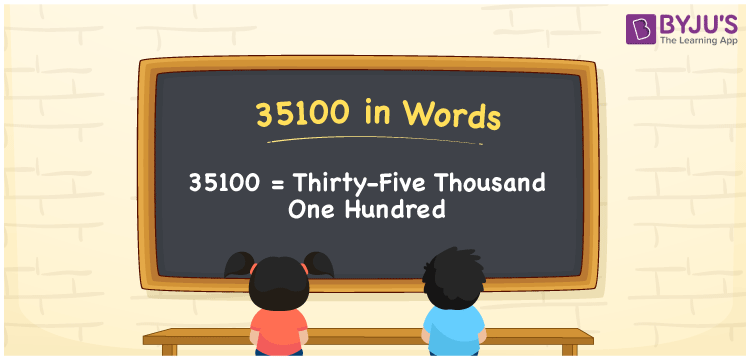# 35100 in Words

Suppose you read a story that contains 35100 words; you can say, “I read a story that has Thirty-five thousand one hundred words”. That means we can communicate 35100 in words as Thirty-five thousand one hundred. In this article, you will learn how to note the cardinal number 35100 in word form with the help of a place value chart.

 35100 in words Thirty-five thousand one hundred Thirty-five thousand one hundred in Numbers 35100

## 35100 in English words

Generally, we can write numbers in words using the English alphabet. So, we can spell 35100 in English as “Thirty-five thousand one hundred”.## How to Write 35100 in Words?

To get the word form of the number 35100, we need to make a place value chart with five columns since it has five digits, as given below.

 Ten thousand Thousands Hundreds Tens Ones 3 5 1 0 0

Here, ones = 0, tens = 0, hundred = 1, thousands = 5, ten thousands = 3

By expanding above digits, we get;

3 × Ten thousand + 5 × Thousand + 1 × Hundred + 0 × Ten + 0 × One

= 3 × 10000 + 5 × 1000 + 1 × 100 + 0 × 10 + 0 × 1

= 30000 + 5000 + 100

= Thirty thousand + Five thousand + One hundred

= Thirty-five thousand one hundred

Therefore, 35100 in words = Thirty-five thousand one hundred.

As we know, 35100 is a natural number that is the successor of 35099 and the predecessor of 35101.

35100 in words – Thirty-five thousand one hundred

Is 35100 an odd number? – No

Is 35100 an even number? – Yes

Is 35100 a perfect square number? – No

Is 35100 a perfect cube number? – No

Is 35100 a prime number? – No

Is 35100 a composite number? – Yes

## Frequently Asked Questions on 35100 in Words

Q1

### How do you spell 35100 in words?

In English words, we can express 35100 as Thirty-five thousand one hundred and thus, we can spell 35100 in words as “Thirty-five thousand one hundred”.
Q2

### How do you write Rs. 35100 in words on a cheque?

On a cheque, we can write an amount of Rs. 35100 in words as Thirty-five thousand one hundred rupees only.
Q3

### What is 35100 in words?

Thirty-five thousand one hundred is the word form of 35100, i.e. 35100 = Thirty-five thousand one hundred.